# 4 Draw a structural formula for each compound. (a) Dimethyl carbonate (b) p-Nitrobenzamide (c) Ethyl 3-hydroxybutanoate (d) Diethyl oxalate (e) Ethyl trans-2-pentenoate (f) Butanoic anhydride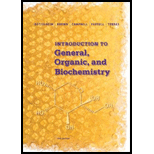### Introduction to General, Organic a...

11th Edition
Frederick A. Bettelheim + 4 others
Publisher: Cengage Learning
ISBN: 9781285869759### Introduction to General, Organic a...

11th Edition
Frederick A. Bettelheim + 4 others
Publisher: Cengage Learning
ISBN: 9781285869759

#### Solutions

Chapter 19, Problem 19.4P
Textbook Problem

## 4 Draw a structural formula for each compound.(a) Dimethyl carbonate(b) p-Nitrobenzamide(c) Ethyl 3-hydroxybutanoate(d) Diethyl oxalate(e) Ethyl trans-2-pentenoate(f) Butanoic anhydride

Expert Solution
Interpretation Introduction

(a)

Interpretation:

To draw the structural formula of given organic compounds.

Concept Introduction:

Ester, amide and anhydride are derivatives of carboxylic acid.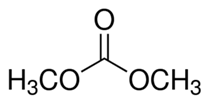### Explanation of Solution

We know that CO3-2 is carbonate ion. When two O atoms of this ion are bonded with methyl group it forms dimethyl carbonate. Hence common name of OC(OCH3 )2 is dimethyl carbonate. The IUPAC name of this compound is Methyl carbonate.

Expert Solution
Interpretation Introduction

(b)

Interpretation:

To draw the structural formula of given organic compounds.

Concept Introduction:

Ester, amide and anhydride are derivatives of carboxylic acid.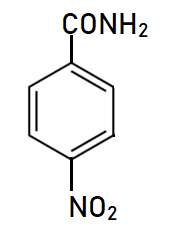### Explanation of Solution

This is a nitro derivative of benzamide in which nitro group is bonded at para position with respect to amide group. Benzamide is C6H5-CONH2 in which the amide group is directly bonded with aromatic ring. Here the nitro-group is bonded at para position.

Expert Solution
Interpretation Introduction

(c)

Interpretation:

To draw the structural formula of given organic compounds.

Concept Introduction:

Ester, amide and anhydride are derivatives of carboxylic acid.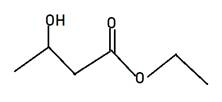### Explanation of Solution

Alkyl alkanoate is the general name for ester. Here Alkyl indicates the small alkyl group in RCOOR and alkanoate is RCOO- part. The name given is ethyl 3-hydroxybutanate, hence 3-hydroxybutanate will be RCOO-part and ethyl will be another r of ester. Therefore, the structure of ethyl 3-hydroxybutanate will be CH3CH(OH)CH2COOCH2CH3.

Expert Solution
Interpretation Introduction

(d)

Interpretation:

To draw the structural formula of given organic compounds.

Concept Introduction:

Ester, amide and anhydride are derivatives of carboxylic acid.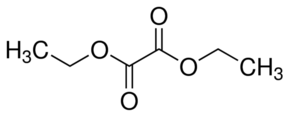### Explanation of Solution

Diethyl oxalate is the diester of oxalic acid (HOOC-COOH). As name suggested, diethyl stands for two ethyl group bonded at both carbon atoms of oxalic acid hence the formula will be C2H5-OCO-COO-C2H5..

Expert Solution
Interpretation Introduction

(e)

Interpretation:

To draw the structural formula of given organic compounds.

Concept Introduction:

Ester, amide and anhydride are derivatives of carboxylic acid.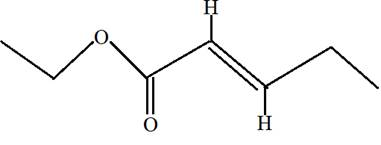Ethyl trans-2-pentenoate.

### Explanation of Solution

Ethyl trans-2-pentenoate is the ester of 2-pentenoic acid with ethanol. Here trans indicates the position of H on both double bonded carbon atoms. Hence in the formula of ester R1 -COO-R2 ; R2 will be ethyl group from alcohol and R1 will be alkyl group from acid.

Expert Solution
Interpretation Introduction

(f)

Interpretation:

To draw the structural formula of given organic compounds.

Concept Introduction:

Ester, amide and anhydride are derivatives of carboxylic acid.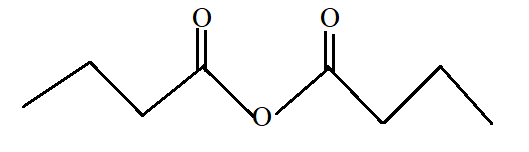Butanoic anhydride.

### Explanation of Solution

Butanoic anhydride is the anhydride of butanoic acid with −COOCO- as functional group. Butanoic acid is CH3-CH2-CH2-COOH. Dehydration or elimination of one water molecule between two butanoic acid forms butanoic anhydride. Hence the structural formula will be CH3-CH2-CH2-COO-CO-CH2-CH2-CH3.

### Want to see more full solutions like this?

Subscribe now to access step-by-step solutions to millions of textbook problems written by subject matter experts!

### Want to see more full solutions like this?

Subscribe now to access step-by-step solutions to millions of textbook problems written by subject matter experts!